NEET  >  NEET Previous Year Questions (2014-22): Electromagnetic Induction

# NEET Previous Year Questions (2014-22): Electromagnetic Induction - Notes | Study Physics Class 12 - NEET

 1 Crore+ students have signed up on EduRev. Have you?

Q.1. A big circular coil of 1000 turns and average radius 10 m is rotating about its horizontal diameter at 2 rad s–1 . If the vertical component of earth’s magnetic field at that place is 2 × 10–5 T and electrical resistance of the coil is 12.56 ohm, then the maximum induced current in the coil will be                (2022)
(1) 1.5 A
(2) 1 A
(3) 2 A
(4) 0.25 A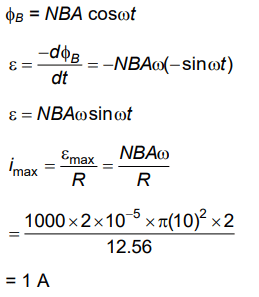Q.2. A square loop of side 1 m and resistance 1Ω is placed in a magnetic field of 0.5 T. If the plane of loop is perpendicular to the direction of magnetic field, the magnetic flux through the loop is
(1) 0.5 weber
(2) 1 weber
(3) Zero weber
(4) 2 weber                  (2022)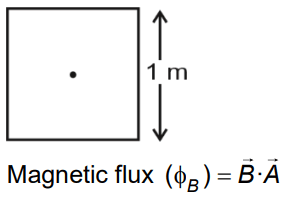Q.3. Two conducting circular loops of radii R1 and Rare placed in the same plane with their centres coinciding. If R1 >> R2, the mutual inductance M between them will be directly proportional to    (2021)
A: R1/R2
B: R2/R1
C: R12/R2
D: R22/R1
Ans:
D
Solution:

Magnetic field at the centre of primary coilConsidering it to be uniform, magnetic flux passing through secondary coil isQ.4. A 800 turn coil of effective area 0.05 m2 is kept perpendicular to a magnetic field 5 × 10–5 T. When the plane of the coil is rotated by 90° around any of its coplanar axis in 0.1 s, the emf induced in the coil will be:    (2019)
A: 2 V
B: 0.2 V
C: 2 x 10-3 V
D: 0.02 V
Ans:
D
Solution:

Magnetic field B = 5 × 10–5 T
Number of turns in coil N = 800
Area of coil A = 0.05 m2
Time taken to rotate Δt = 0.1 s
Initial angle θ1 = 0°
Final angle θ2 = 90°
Change in magnetic flux Δφ
= NBAcos90° – BAcos0°
= – NBA
= – 800 × 5 × 10–5 × 0.05
= – 2 × 10–3 weber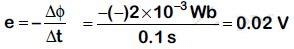Q.5. In which of the following devices, the eddy current effect is not used?    (2019)
A: Induction furnace
B: Magnetic braking in train
C: Electromagnet
D: Electric heater
Ans: D
Solution:

Electric heater does not involve Eddy currents. It uses Joule's heating effect.

Q.6. A metallic rod of mass per unit length 0.5 kg m–1 is lying horizontally on a smooth inclined plane which makes an angle of 30° with the horizontal. The rod is not allowed to slide down by flowing a current through it when a magnetic field of induction 0.25T is acting on it in the vertical direction. The current flowing in the rod to keep is stationary is    (2018)
A: 7.14 A
B: 5.98 A
C: 14.76 A
D: 11.32 A
Ans:
D
Solution: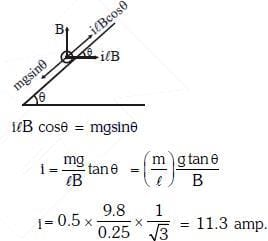Q.7. A thin diamagnetic rod is placed vertically between the poles of an electro-magnet. When the current in the electro-magnet is switched on, then the diamagnetic rod is pushed up, out of the horizontal magnetic field. Hence the rod gains gravitational potential energy. The work required to do this comes from     (2018)
A: the current source
B: the magnetic field
C: the lattice structure of the material of the rod
D: the induced electric field due to the changing magnetic field
Ans:
A
Solution:

When current source is switched on, magnetic field sets up between poles on electromagnet. Diamagnetic material, due to its tendency to move from stronger to weaker field, is thus repelled out.

Q.8. A long solenoid of diameter 0.1 m has 2 × 104 turns per meter. At the centre of the solenoid, a coil of 100 turns and radius 0.01 m is placed with its axis coinciding with the solenoid axis. The current in the solenoid reduces at a constant rate to 0 A from 4 A in 0.05 s. If the resistance of the coil is 10π2Ω. The total charge flowing through the coil during this time is :-    (2017)
A: 16 μC
B: 32 μC
C: 16 π μC

D: 32 π μC
Ans: B
Solution: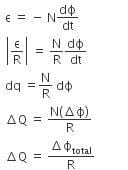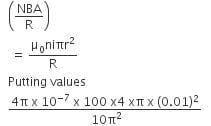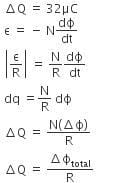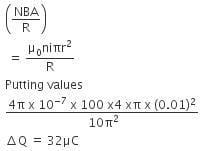Q.9. A long solenoid has 1000 turns. When a current of 4A flows through it, the magnetic flux linked with each turn of the solenoid is 4 x 10-3 Wb. The self-inductance of the solenoid is    (2016)
A: 1H
B: 4H
C: 3H
D: 2H
Ans:
A
Solution:

Given,
Number of turns in the solenoid, N = 1000
Current, I = 4 A
Magnetic flux, straight phi subscript straight B = 4 x 10-3 Wb
Self-inductance of solenoid is given by,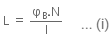Putting the value of equation in (i), we get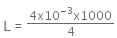= 1H

Q.10. A conducting square frame of side 'a‘ and a long straight wire carrying current I are located in the same plane as shown in the figure. The frame moves to the right with a constant velocity 'V‘. The emf induced in the frame will be proportional to :     (2015)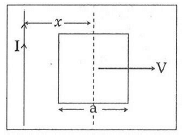A: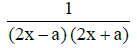B: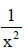C: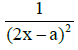D: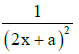Ans: A
Solution: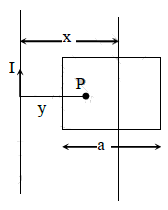See figure alongside.
Let x be the distance of the centre of the frame from the long straight wire carrying current I.
Consider the point P at a distance y from the long straight wire carrying current I.
Strength of magnetic induction at point P is given by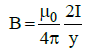Integrating over y from y = (x - a/2) to y = (x + a/2)
We get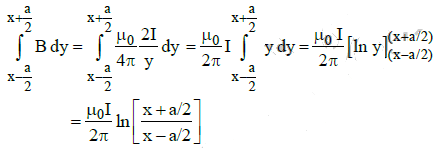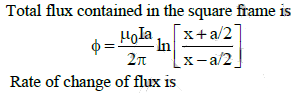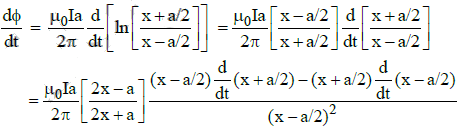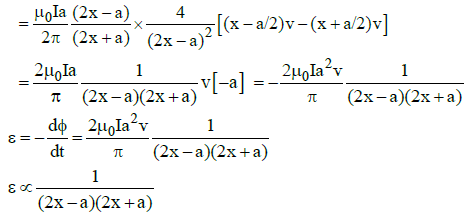Q.11. A thin semicircular conducting ring (PQR) of radius ‘r’ is falling with its plane vertical in a horizontal magnetic field B, as shown in figure. The potential difference developed across the ring when its speed is v, is :    (2014)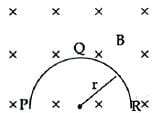A: πrBv and R is at higher potential
B: 2rBv and R is at higher potential
C: Zero
D: Bvπr2/2 & P is at higher potential
An:
B
Solution:

Effective length = 2r
Potential developed = 2rBv

The document NEET Previous Year Questions (2014-22): Electromagnetic Induction - Notes | Study Physics Class 12 - NEET is a part of the NEET Course Physics Class 12.
All you need of NEET at this link: NEET

## Physics Class 12

157 videos|452 docs|213 tests

## Physics Class 12

157 videos|452 docs|213 tests

### How to Prepare for NEET

Read our guide to prepare for NEET which is created by Toppers & the best Teachers

Track your progress, build streaks, highlight & save important lessons and more!

,

,

,

,

,

,

,

,

,

,

,

,

,

,

,

,

,

,

,

,

,

;